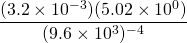Chapter 6: Polynomials

# 6.3 Scientific Notation (Homework Assignment)

Scientific notation is a convenient notation system used to represent large and small numbers. Examples of these are the mass of the sun or the mass of an electron in kilograms. Simplifying basic operations such as multiplication and division with these numbers requires using exponential properties.

Scientific notation has two parts: a number between one and nine and a power of ten, by which that number is multiplied.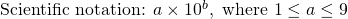The exponent tells how many times to multiply by 10. Each multiple of 10 shifts the decimal point one place. To decide which direction to move the decimal (left or right), recall that positive exponents means there is big number (larger than ten) and negative exponents means there is a small number (less than one).

Example 6.3.1

Convert 14,200 to scientific notation.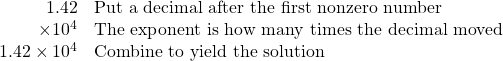Example 6.3.2

Convert 0.0028 to scientific notation.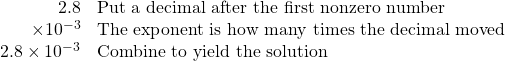Example 6.3.3

Convert 3.21 × 105 to standard notation.

Starting with 3.21, Shift the decimal 5 places to the right, or multiply 3.21 by 105.

321,000 is the solution.

Example 6.3.4

Convert 7.4 × 10−3 to standard notation

Shift the decimal 3 places to the left, or divide 6.4 by 103.

0.0074 is the solution.

Working with scientific notation is easier than working with other exponential notation, since the base of the exponent is always 10. This means that the exponents can be treated separately from any other numbers. For instance:

Example 6.3.5

Multiply (2.1 × 10−7)(3.7 × 105).

First, multiply the numbers 2.1 and 3.7, which equals 7.77.

Second, use the product rule of exponents to simplify the expression 10−7 × 105, which yields 10−2.

Combine these terms to yield the solution 7.77 × 10−2.

Example 6.3.6

(4.96 × 104) ÷ (3.1 × 10−3)

First, divide: 4.96 ÷ 3.1 = 1.6

Second, subtract the exponents (it is a division): 104− −3  = 104 + 3  = 107

Combine these to yield the solution 1.6 × 107.

# Questions

For questions 1 to 6, write each number in scientific notation.

1. 885.3
2. 0.000744
3. 0.081
4. 1.09
5. 0.039
6. 15,000

For questions 7 to 12, write each number in standard notation.

1. 8.7 × 105
2. 2.56 × 102
3. 9 × 10−4
4. 5 × 104
5. 2 × 100
6. 6 × 10−5

For questions 13 to 20, simplify each expression and write each answer in scientific notation.

1. (7 × 10−1)(2 × 10−3)
2. (2 × 10−6)(8.8 × 10−5)
3. (5.26 × 10−5)(3.16 × 10−2)
4. (5.1 × 106)(9.84 × 10−1)
5.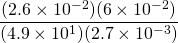6.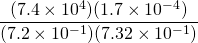7.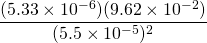8.